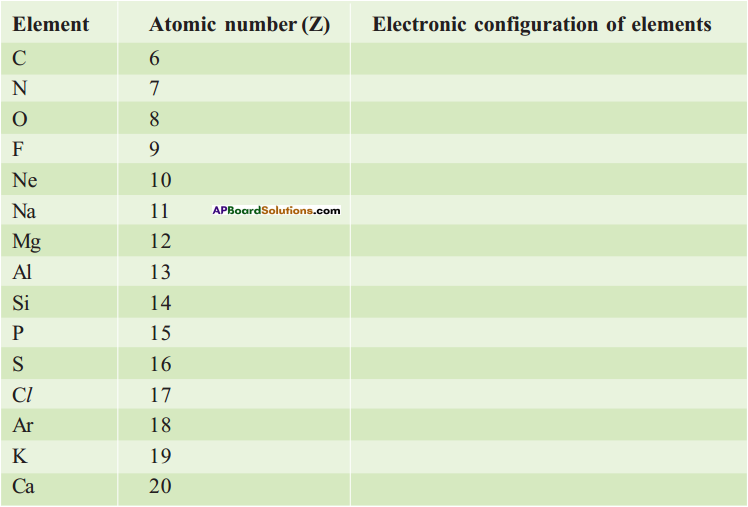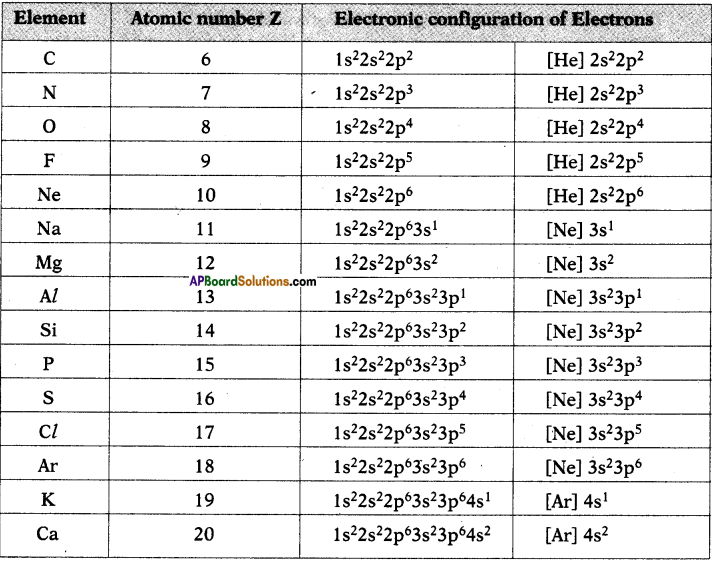# AP SSC 10th Class Chemistry Solutions Chapter 8 Structure of Atom

AP State Board Syllabus AP SSC 10th Class Chemistry Solutions Chapter 8 Structure of Atom Textbook Questions and Answers.

## AP State Syllabus SSC 10th Class Chemistry Solutions 8th Lesson Structure of Atom

### 10th Class Chemistry 8th Lesson Structure of Atom Textbook Questions and Answers

Question 1.
What information does the electronic configuration of an atom provide? (AS1)

• The distribution of electrons in shells, sub-shells and orbital in an atom is known as electronic configuration.
• It provides the information of position of an electron in the space of atom.
• The distribution of electrons in various atomic orbitals provides an understanding of the electronic behaviour of the atom and in turn its reactivity.
• The short hand notation is as shown below.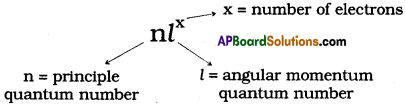Question 2.
a) How many maximum number of electrons that can be accommodated in a principal energy shell?
The maximum number of electrons that can be accommodated in a principal energy shell is 2n². Here n is principal quantum number.

b) How many maximum number of electrons that can be accommodated in a sub-shell?
The maximum number of electrons that can be accommodated in a sub-shell is 2(2l +1) (where l is orbital quantum number).

c) How many maximum number of electrons can that be accommodated in an orbital?
The maximum number of electrons that can be accommodated in an orbital is 2.

d) How many sub-shells are present in a principal energy shell?
The number of sub-shells in a principal energy shell is n (n is principal quantum number).

e) How many spin orientations are possible for an electron in an orbital?
Answeer:
The spin orientations possible for an electron in an orbital are 2.Question 3.
In an atom the number of electrons in M-shell is equal to the number of electrons in the K and L-shell. Answer the following questions. (AS1)
a) Which is the outermost shell?
The outermost shell is N shell.

b) How many electrons are there in its outermost shell?
Two electrons are there in outermost shell.

c) What is the atomic number of element?
The atomic number of element is 22.

d) Write the electronic configuration of the element.
The element is Ti (Titanium). Its electronic configuration is 1s²2s²2p63s²3p64s²3d².

Reason :

• Electrons enter M shell after completion of K and L.
• So the number of electrons in M shell is 10.
• But after completion of 3p orbital electron enters 4s before entering to 3d.
• So outermost orbit or shell is N shell.
• So the atomic number of element is 22.
• Its electron configuration is 1s² 2s² 2p6 3s² 3p6 4s² 3d².

Question 4.
Rainbow is an example for continuous spectrum – explain. (AS1)
(OR)
Which is naturally occurring continuous spectrum ? Explain.

• Rainbow is a spectrum of different colours (VIBGYOR) with different wavelengths.
• These colours are continuously distributed.
• There is no fixed boundary for each colour.
• Hence, rainbow is a continuous spectrum.Question 5.
How many elliptical orbits are added by Sommerfeld in third Bohr’s orbit ? What was the purpose of adding these elliptical orbits? (AS1)
Sommerfeld added two elliptical orbits to Bohr’s third orbit.

Purpose of adding elliptical orbits :

• Bohr’s model failed to account for splitting of line spectra and line spectrum.
• In an attempt to account for the structure of line spectrum, Sommerfeld modified Bohr’s atomic model by adding elliptical orbits.

Question 6.
What is absorption spectrum?
Absorption spectrum: The spectrum formed by the absorption of energy when electron jumps from lower energy level to higher energy level is called absorption spectrum. It contains dark lines on bright background.

Question 7.
What is an orbital? How it is different from Bohr’s orbit? (AS1)
(OR)
Comparison between orbit and orbital.
The region of space around the nucleus where the probability of finding electron is maximum is called orbital. Whereas orbit is the path of the electron around the nucleus.

These two are differentiated like this.

 Orbit Orbital 1. Path of electron around nucleus. 1) Probability of finding electron around nucleus. 2. Orbits are represented by letters K, L, M, N, 0, …….etc. 2. Orbitals are represented by letters s, p, d, f, g, …….etc. 3) Its information is given by principal 3) Its information is given by orbital quantum number. 4) It is two dimensional. 4) It is three dimensional. 5) It does not satisfy Heisenberg’s uncertainty principle. 5) It satisfies the Heisenberg’s principle of uncertainty.

Question 8.
Explain the significance of three quantum numbers in predicting the positions of an electron in an orbit. (AS1)
(OR)
How are quantum numbers helpful to understand the atomic structure?
Significance of three quantum numbers in predicting the positions of an electron in an orbit.

1) Principal quantum number (n) :
The principal quantum number explains about the size and energy of shells (or) orbitals. It is denoted by n.

As ‘n’ increases, the orbitals become larger and the electrons in those orbitals are farther from the nucleus.

It takes values 1, 2, 3, 4, ……………. for that the shells are represented by letters K, L, M, N, ……….

The number of electrons in a shell is limited to 2n².

2) The Angular – momentum quantum number (l) :
The angular momentum quantum number defines the shape of the orbital occupied by the electron and the orbital angular momentum of the electron, is in motion.

l takes values from 0 to n – 1 for these values the orbitals are designated by letters s, p, d, f, ………….. etc.

l also governs the degree with which the electron is attached to nucleus. The larger the value of l, the smaller is the bond with which it is maintained with the nucleus.

3) Magnetic orbital quantum number (ml) :
The orientation of orbital with external magnetic field determines magnetic orbital quantum number.

ml has integer values between – l and l including zero.

The number of values for m, are 2l + l, which give the number of orbitals per sub-shell. The maximum number of electrons in orbitals in the sub-shell is 2 (2l + l).Question 9.
What is nlx method? How is it useful? (AS1)
(OR)
What is nlx method? How is it useful in electronic configuration?
The shorthand notation consists of the principal energy level (n value) the letter representing sub – level (l value), and the number of electrons (x) in the sub-shell is written as superscript nlx.

It is useful in writing electron configuration of elements. For example, in Hydrogen (H), the set of quantum numbers is n = 1, l = 0, ml = 0, ms = ½ or – ½. The electronic configuration is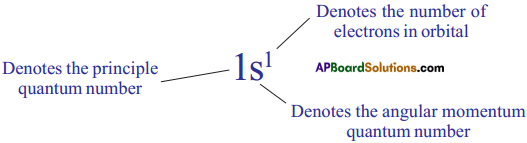Question 10.
Following orbital diagram shows the electronic configuration of nitrogen atom. Which rule does not support this? (AS1)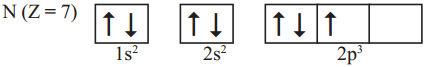(OR)
Write the correct electronic configuration of the given nitrogen atom with the help of Hund’s rule.• This electron configuration does not support Hund’s rule.
• According to Hund’s rule, the orbitals of equal energy are occupied with one elec-tron each before pairing of electrons starts.
• Here, pairing of electrons in 2px orbital was taken place without filling of an elec-tron in 2pz orbital.
• Hence the correct electron configuration is as follows.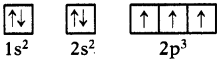Question 11.
Which rule is violated in the electronic configuration 1s0 2s² 2p4?

• Aufbau principle is violated in this electronic configuration because according to Aufbau principle, electron enters orbital of lowest energy.
• Among 1s, 2s and 2p, Is has least energy.
• So Is orbital must be filled before the electron should enter 2s.Question 12.
Write the four quantum numbers for the differentiating electron of sodium (Na) atom. (AS1)
The electronic configuration of sodium (Na) is 1s² 2s² 2p6 3s¹. So the differentiating electron enters 3s. Therefore the four quantum numbers are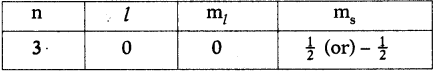Question 13.
What is emission spectrum?
(OR)
When radiation is emitted what is the name given to such spectrum? Explain such spectrum.

• The spectrum produced by the emitted radiation is known as emission spectrum.
• This spectrum corresponds to liberation of energy when an excited electron returns back to ground state.

Emission spectrum is of two types :

1) Continuous spectrum :
When white light passes through a prism it dissociates into seven colours. This spectrum is called continuous spectrum.

2) Discontinuous spectrum :
Discontinuous spectrum is of two types.

a) Line spectrum :
The spectrum with sharp and distinct lines. It is given by gaseous atoms.

b) Band spectrum :
The spectrum very closely spaced lines is known as band spectrum. It is given by molecule.

Question 14.
i) An electron in an atom has the following set of four quantum numbers to which orbital it belong to : (AS2)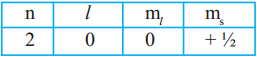This electron belongs to 2s orbital.
Spin is in clockwise direction. ⇒ 2s¹

ii) Write the fojur quantum numbers for Is1 electron. (AS1)
The four quantum numbers for Is1 electron are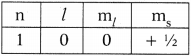Question 15.
Which electronic shell is at a higher energy level K or L? (AS2)
L – shell is at higher energy level, because it is far from nucleus than K shell.

Question 16.
Collect the information regarding wavelengths and corresponding frequencies of three primary colours red, blue and green. (AS4)
The wavelengths and corresponding frequencies of three primary colours red, blue and green are given below.

 Primary colours Wavelength in nm (1 nm = 10-9m) Frequency in Hz (Hertz) Red 700 4.29 × 1014 Green 530 5.66 × 1014 Blue 470 6.38 × 1014

Question 17.
The wavelength of a radio wave is 1.0 m. Find its frequency. (AS7)
c = 3 × 108 m/s ; λ = 1m ; c = vλ ⇒ v = $$\frac{\mathrm{c}}{\lambda}=\frac{3 \times 10^{8}}{1}$$ = 3 × 108 Hz.Question 18.
Why are there exemptions in writing the electronic configurations of Chromium and Copper?
1. Elements which have half-filled or completely filled orbitals have greater stability.

2. So in chromium and copper the electrons in 4s and 3d redistributes their energies to attain stability by acquiring half-filled and completely filled d-orbitals.

3. Hence the actual electronic configuration of chromium and copper are as follows.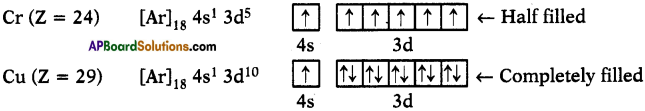Fill In The Blanks

1. If n = 1, then angular momention quantum number (l) = …………………
2. If a sub-shell is denoted as 2p, then its magnetic quantum number values are …………………, …………………, …………………
3. Maximum number of electrons that an M-shell contain is / are …………………
4. For ‘n’, the minimum value is ………………… and the maximum value is …………………
5. For?, the minimum value is ………………… and the maximum value is …………………
6. For’m/ the minimum value is ………………… and the maximum value is …………………
7. The value of ‘ms’ for an electron spinning in clockwise direction is ………………… and for anti-clockwise direction is …………………

1. 0
2. – 1, 0, + 1
3. 18
4. 1, – ∞
5. 0, (n – 1)
6. – l, + l
7. + ½, – ½

Multiple Choice Questions

1. An emission spectrum consists of bright spectral lines on a dark back ground. Which one of the following does not correspond to the bright spectral lines?
D) Velocity of light
D) Velocity of light

2. The maximum number of electrons that can be accommodated in the L-shell of an atom is
A) 2
B) 4
C) 8
D) 16
C) 83. If l = 1 for an atom, then the number of orbitals in its sub-shell is
A) 1
B) 2
C) 3
D) 0
C) 3

4. The quantum number which explains about size and energy of the orbit or shell is
A) n
B) l
C) ml
D) ms
A) n

### 10th Class Chemistry 8th Lesson Structure of Atom InText Questions and Answers

10th Class Chemistry Textbook Page No. 112

Question 1.
How many colours are there in a rainbow? What are they?
There are seven colours in a rainbow. They are Violet, Indigo, Blue, Green, Yellow,Orange and Red.

Question 2.
What are the characteristics of electromagnetic waves?
A.nswer:
Electromagnetic energy is characterised by wavelength (l) and frequency (u).

10th Class Chemistry Textbook Page No. 113

Question 3.
Can we apply this equation c = uA, to a sound wave?
Yes. It is a universal relationship and applies to all waves.

10th Class Chemistry Textbook Page No. 114

Question 4.
What happens when you heat an iron rod on a flame? Do you find any change in colour while heating an iron rod?

• When we heat an iron rod, some amount of heat energy that was absorbed by iron rod is emitted as light.
• First iron turns into red (lower energy corresponding to higher wavelength) and as the temperature rises it glows and turns into orange, yellow, blue or even white respectively (higher energy and lower wavelength).
• If we go on heating the rod, it turns into white light which includes all visible wavelengths.
• So we find some changes in colour while heating an iron rod.

Question 5.
Do you observe any other colour at the same time when one colour is emitted?
While heating the rod if the temperature is high enough, other colours will also be emitted, but due to higher intensity of one particular emitted colour (eg.: red), others cannot be observed.Question 6.
How do various colours come from fire works?
(OR)
Do you enjoy Deepavali fire works? Variety of colours is seen from fire works. How do these colours come from fire works?
Yes. The electrons present in atoms of elements absorb energy and move to excited states and they return to ground state with emission of energy in visible spectrum. So the colours observed during fire works are the emitted energy by various elements in different fire works.

10th Class Chemistry Textbook Page No. 115

Question 7.
Do you observe yellow light in street lamps? Which will produce yellow light?
Yes, sodium vapours produce yellow light in street lamps.

Question 8.
Why do different elements emit different flame colours when heated by the same non-luminous flame?

• All the materials are made up of atoms and molecules. These atoms and molecules possess certain fixed energy.
• An atom or molecule having lowest possible energy is said to be in ground state.
• When we heat the materials the electrons of these atoms gain energy and move to excited states (higher energy state).
• An atom of molecule in excited state can emit light to lower its energy in order to get stability and come back to ground state.
• Light emitted in such process has certain fixed wavelength for one kind of atoms.
• The light emitted by different kinds of atoms is different because the excited states electrons will go are different. So different elements produce different flame colours.

Question 9.
What happens when an electron gains energy?
The electron moves to higher energy level called the excited state.

10th Class Chemistry Textbook Page No. 116

Question 10.
Does the electron retain the energy forever?
The electron loses the energy and comes back to its ground state. The energy emitted by the electron is seen in the form of electromagnetic energy.Question 11.
Did Bohr’s model account for the splitting of line spectra of a hydrogen atom into finer lines?
No, Bohr’s model failed to account for splitting of line spectra.

Question 12.
Why is the electron in an atom restricted to revolve around the nucleus at certain fixed distances?
In order to explain the atomic spectra, Bohr-Sommerfeld model proposed that the electrons are restricted to revolve around the nucleus at certain fixed distances.

10th Class Chemistry Textbook Page No. 117

Question 13.
Do the electrons follow defined paths around the nucleus?
No, they revolve around the nucleus in a region called orbital.

Question 14.
What is the velocity of the electron?
It is very close to light.Question 15.
Is it possible to find exact position of electron? How do you find the position and velocity of an electron?
No, as the electrons are very small, light of very short wavelength is required for this task.

This short wavelength light interacts with the electron and disturbs the motion of electron. So it is not possible to find exact position and velocity of electron simultaneously. Whereas we can find the region where the probability of finding electron is more.

Question 16.
Do atoms have a definite boundary, as suggested by Bohr’s model?
Yes, atoms have definite boundary.

Question 17.
What do we call the region of space where the electron might be, at a given time?
The region of space around the nucleus where the probability of finding an electron is maximum, called an orbital.

10th Class Chemistry Textbook Page No. 118

Question 18.
What information do the quantum numbers provide?
The quantum numbers describe the space around the nucleus where the electrons are found and also their energies.

Question 19.
What does each quantum number signify?
The quantum numbers signify the probability of finding electron in the space around nucleus.

10th Class Chemistry Textbook Page No. 119

Question 20.
What is the maximum value of/for n = 4?
The maximum value of / for n = 4 is 3.Question 21.
How many values can l have for n = 4?
l takes values from 0 to n – 1. So l has 4 values for n = 4. Those values are 0, 1,2, 3.

Question 22.
Do all the p-orbitals have the same energy? A. Orbitals in the sub-shell belonging to same shell possess same energy but they differ in their orientations.

10th Class Chemistry Textbook Page No. 121

Question 23.
How are two electrons in the Helium atom arranged?
They are arranged in pair in Is orbital and the electronic configuration is 1s².

10th Class Chemistry Textbook Page No. 122

Question 24.
What are the spins of two electrons in an orbital?
The two electrons in an orbital have opposite spins. If one is clockwise spin, then other electron has anti-clockwise spin.Question 25.
How many electrons can occupy an orbital?
An orbital can hold only two electrons.

10th Class Chemistry 8th Lesson Structure of Atom Activities

Activity – 1

Question 1.
Explain the wave nature of light.
(OR)
How does light behave? Explain.

• Light is an electromagnetic wave.
• Electromagnetic waves are produced when an electric charge vibrates.
• This vibrating electric charge creates a change in the electric field. The changing electric field creates a changing magnetic field.
• This process continues with both the created fields being perpendicular to each other and at right angles to the direction of propagation of the wave.
• This electromagnetic wave is produced.

Activity – 2

Question 2.
Write an activity which shows metal produces colour in flame.
(OR)
‘Metal produces colour in a flame.’ Prove the statement by giving examples.
A)

• Take a pinch of cupric chloride in a watch glass and make a paste with concentrated hydrochloric acid.
• Take this paste on a platinum loop and introduce it into a non-luminous flame.
• Cupric chloride produces a green colour flame.

B)

• Take a pinch of strontium chloride in a watch glass and make a paste with concentrated hydrochloric acid.
• Take this paste on a platinum loop and introduce it into a non-luminous flame.
• Strontium chloride produces a crimson red flame.

Activity – 3

Question 3.
Complete the electronic configuration of the following elements.